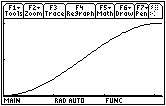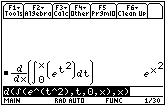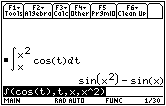## Lesson 1

Lesson 1
16.1.1   By the pattern shown, the area under the curve between x = 0 and x = 5 should be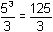, which is confirmed by the TI-89.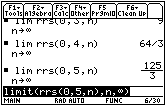16.1.2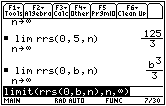The area under the curve between x = 0 and x = b is given by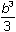.

16.1.3
Area Function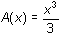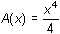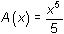Curve Function
f(x) = x2
f(x) = x3
f(x) = x4

16.1.4   The derivative of the area function is the curve function.

Lesson 2
Lesson 2

16.2.1 Evaluate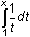for each value of x by using the integral key and entering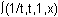.

x
1.0
1.5
2.0
2.5
3.0
3.5
4.0
area
0
0.405
0.693
0.916
1.099
1.253
1.386

16.2.2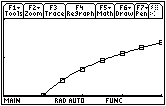The regression equation is approximately y = ln x

16.2.3= ln x

16.2.4   The derivative of the area function, A(x) = ln x, is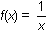, the curve function.

16.2.5   The height of the curve is increasing, so the slopes of the area function are increasing and its graph is concave upward. An approximate area function graph is shown below. The actual values of the area function are not as important as the graph's shape.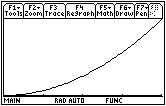Lesson 3
Lesson 3

16.3.1 Predict:

1.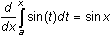2.

3.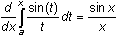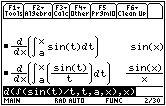16.3.2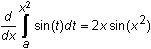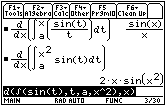Notice that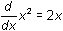appears in the result, 2x sin(x2) .

16.3.3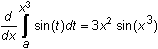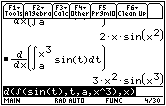16.3.4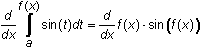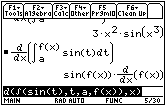16.3.5 Because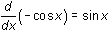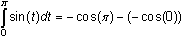= –(–1) + 1

= 2

16.3.6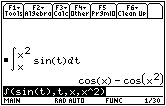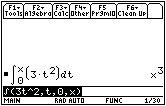The derivative of the area function with respect to x is f(x).